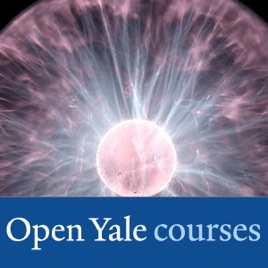25 episodes

This is a continuation of the introductory course on the principles and methods of physics for students who have good preparation in physics and mathematics. This course covers electricity, magnetism, optics and quantum mechanics.# Fundamentals of Physics, II - Video Yale University

• Science

This is a continuation of the introductory course on the principles and methods of physics for students who have good preparation in physics and mathematics. This course covers electricity, magnetism, optics and quantum mechanics.

• video
01 – Electrostatics

## 01 – Electrostatics

The second half of the course begins with a discussion of electricity. The concept of charge is introduced, and the properties of electrical forces are compared with those of other familiar forces, such as gravitation. Coulomb's Law, along with the principle of superposition, allows for the calculation of electrostatic forces from a given charge distribution.

• 1 hr 6 min
• video
02 - Electric Fields

## 02 - Electric Fields

The electric field is introduced as the mediator of electrostatic interactions: objects generate the field which permeates all of space, and charged objects in the field experience a force with magnitude proportional to their charge. Several instructive examples are given, including the field of an electric dipole and the notion of the electric dipole and dipole moment. The notion of field lines is introduced.

• 1 hr 13 min
• video
03 - Gauss's Law I

## 03 - Gauss's Law I

The electric field is discussed in greater detail and field due an infinite line charge is computed. The concepts of charge density and electric flux are introduced and Gauss's Law, which relates the two, is derived. It is applied to the study of the electric field generated by a spherical charge distribution.

• 1 hr 11 min
• video
04 - Gauss's Law and Application to Conductors and Insulators

## 04 - Gauss's Law and Application to Conductors and Insulators

Lecture begins with a recap of Gauss's Law, its derivation, its limitation and its applications in deriving the electric field of several symmetric geometries—like the infinitely long wire. The electrical properties of conductors and insulators are discussed. Multiple integrals are briefly reviewed.

• 1 hr 15 min
• video
05 - The Electric Potential and Conservation of Energy

## 05 - The Electric Potential and Conservation of Energy

The law of conservation of energy is reviewed using examples drawn from Newtonian mechanics. The work-energy theorem is derived from first principles and used to initiate a discussion of the vector calculus underlying the law of conservation of energy.

• 1 hr 14 min
• video
06 - Capacitors

## 06 - Capacitors

The electric potential is defined for the electric field. It is introduced as an integral of the electric field making the field the derivative of the potential. After discussing the ideas of electric potential and field as presented in the previous lecture, the concept of capacitance is introduced as a means of storing charge and energy.

• 1 hr 12 min

## Customer Reviews

4.3 out of 5
11 Ratings

11 Ratings

Stanzer ,

### Videos don

When I try accessing the video lectures, for some reason, the videos don't play at all. In fact, none of the videos do.